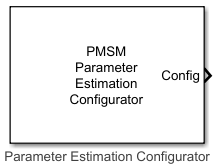# PMSM Parameter Estimation Configurator

Generate configuration elements for PMSM parameter estimation

Since R2023a

•Libraries:
Motor Control Blockset / Parameter Estimation / PMSM Parameter Estimation

## Description

The PMSM Parameter Estimation Configurator block generates the following configuration elements for estimating the parameters of a permanent magnet synchronous motor (PMSM):

• Elements for motor and inverter hardware

• Elements for estimating motor resistance (Rs)

• Elements for estimating motor inductances (Ld and Lq)

• Elements for estimating back EMF constant (Ke), motor inertia (J), and friction constant (B)

The block uses the parameter settings to generate an output bus containing the configuration elements. You can use the configuration elements in these PMSM parameter estimation blocks:

## Ports

### Output

expand all

The bus signal includes these configuration elements.

CategoryConfiguration elementUnits

Elements for motor and inverter hardware

Number of pole pairs

-

Rated speed of motor

RPM

Rated current of motor

A

Maximum measurable inverter current

A

Board resistance

ohm

Current sense gain

Voltage sense gain

Parameter estimation sample time

seconds

Elements for estimating motor inductances (Ld and Lq)

Maximum frequency

Hz

Minimum frequency

Hz

Step for frequency sweep

Hz

Frequency step size for estimation

Hz

Number of frequencies for estimation

-

Elements for estimating back EMF constant (Ke), motor inertia (J), and friction constant (B)

Back EMF estimation time

seconds

Back EMF estimation speed

RPM

Specify current controller gains (off/on)

-

Proportional gain

-

Integral gain

-

Iq Reference current for FOC

PU current

Lower speed limit for inertia estimation

RPM

The configuration elements are analogous to the block parameters. For more information about these elements, see Parameters.

Data Types: `single`

## Parameters

expand all

Motor and Inverter

Number of pole pairs available in the PMSM.

Rated speed of the PMSM (in RPM).

Rated current of the PMSM (in Amperes).

Maximum current (in Amperes) that the system (including inverter and controller) can measure.

Resistance in the path of phase currents (excluding motor resistance). You can obtain this value from the datasheet of the inverter hardware.

Gain of the current sense circuit of the inverter. This value determines the current (in Amperes) corresponding to one analog-to-digital converter (ADC) count (resolution of the measurable current range).

Gain of the voltage sense circuit of the inverter. This value determines the voltage (in Volts) corresponding to one analog-to-digital converter (ADC) count (resolution of the measurable voltage range).

Ld and Lq Estimation

Maximum frequency (in Hertz) injected by the Ld Estimator and Lq Estimator blocks to begin the frequency sweep. For more details, see Ld Estimator and Lq Estimator.

Minimum frequency (in Hertz) injected by the Ld Estimator and Lq Estimator blocks to conclude the frequency sweep. For more details, see Ld Estimator and Lq Estimator.

Step size (in Hertz) using which the Ld Estimator and Lq Estimator blocks decrement the frequency during the frequency sweep. For more details, see Ld Estimator and Lq Estimator.

Step size (in Hertz) using which the Ld Estimator and Lq Estimator blocks increment the frequency during the Ld and Lq estimation process. For more details, see Ld Estimator and Lq Estimator.

Number of frequencies (with a step size defined by the Frequency step size for estimation (Hz) parameter) that the Ld Estimator and Lq Estimator blocks inject after the frequency sweep to estimate Ld and Lq parameters. For more details, see Ld Estimator and Lq Estimator.

Ke, J, B Estimation

Duration (in seconds) for which the PMSM Mechanical Parameter Estimator block runs the motor using closed-loop control to estimate back-EMF, Ke. For more details, see PMSM Mechanical Parameter Estimator.

Speed (in RPM) at which the PMSM Mechanical Parameter Estimator block runs the motor using closed-loop control to estimate back-EMF, Ke. For more details, see PMSM Mechanical Parameter Estimator.

Reference current, Iq, to achieve the reference speed specified in the Back EMF estimation speed (RPM) parameter. For more details, see PMSM Mechanical Parameter Estimator.

Select this parameter to enable these fields:

• Proportional gain

• Integral gain

You can use these fields to enter the proportional and integral gains of the PI current controllers that implement closed-loop control to estimate Ke, J, and B.

If you clear this parameter, the block automatically computes the proportional and integral gains.

Proportional gain, kp, of the PI current controller that implements field-oriented control to run the motor in closed-loop control and estimate Ke, J, and B.

#### Dependencies

To enable this parameter, select the Specify current controller gains parameter.

Integral gain, ki, of the PI current controller that implements field-oriented control to run the motor in closed-loop control and estimate Ke, J, and B.

#### Dependencies

To enable this parameter, select the Specify current controller gains parameter.

Lower speed limit during coast-down at which the PMSM Mechanical Parameter Estimation block registers the timestamp for estimating motor inertia. For more information about the estimation process, see PMSM Mechanical Parameter Estimator.

Sample time for the parameter estimation algorithm. This parameter defines the sample time in these parameter estimation blocks for PMSMs:

• PMSM Rs Estimator

• Ld Estimator

• Lq Estimator

• PMSM Mechanical Parameter Estimator

## Version History

Introduced in R2023a